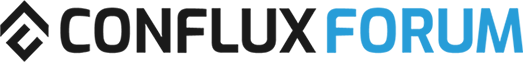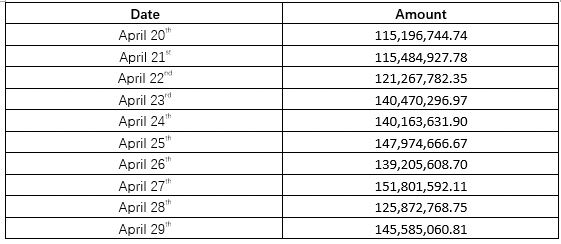# Swappi Second Inject CFX Amount Calculation

Based on rules that describe here: Liquidity Incentive Program for Swappi

``````How to determine the injected CFX amount?
``````

We will monitor the TVL growth of Swappi of different assets and use the combination of the following two rules to determine the injected CFX amount.

For every 10 days, we will calculate the minimum amount of the locked CFX amount in Swappi protocol over the period. Suppose this minimum 10-day locked amount is X. Then ecosystem fund will inject 8% * X CFX in total. Note that this rule will only apply one-time incrementally.

And first injection CFX amount calculation follow that rule, here: Liquidity Incentive Program for Swappi

The above transactions are based on the “How to determine the injected CFX amount” item 1. From Apr. 10th to Apr. 19th the total locked CFX amount is 63,384,900CFX, therefore the Conflux Foundation injected 63384900CFX x 8% = 5,070,792CFX

But not same at second injection CFX amount calculation: Liquidity Incentive Program for SwappiFrom Apr. 20th to Apr. 29th the total locked CFX incremental amount is 115,196,744.74 (20th) - 63,384,900 (10th) = 51,811,844CFX, therefore the Conflux Foundation injected 51,811,844 x 8% = 4,144,947.52 CFX.

Q1: If we look on second schedule. In range from 20th till 29th April minimal amount of CFX locked or X =115,196,744.74 CFX (20th April).
So, 115196744.74 CFX * 8% = 9 215 739,52 CFX must be injected or im wrong here?.
If we would be calculated in same manner as:
(Xn - Xn-1) * 8%, we can get situation when Xn < X n-1 and result would be “ - CFX “ . And here no any meaning of same formula in Rules.
As example: Xn (minimal CFX locked amount from 29th April till 8th May would be 100 000 000 CFX or 100 CFX no matter), Xn-1 = 115 196 744,74 CFX.
If we calculated, as Conflux Foundation new one way accepted manner, we must : (100 000 000 - 115 196 744,74) *8% = -15 196 744,74 * 8% = −1 215 739,52 CFX it’s how much Foundation must send back to Ecosystem Fund immediately from LP Swappi pool. Right?
Q2: Also, why they doubled days in ranges: 10 - 20 April ( include 20 April) , 20 - 29 April (again include 20 April). Correct ranging not doubled days: 20 - 29 April , next range 30th April - 9 May , no problems.

Q3(based on quote from here: For every 30 days, we will calculate the weighted value of all locked assets and inject CFX equal to 1% of the weighted value. This rule will apply recurringly every 30 days. The weighted value is calculated as:
Total locked CFX value + 20% * Total locked other asset value

Plz exact what means every 30 days? 30 days since 10th April = 3 full injection range till 9th May = 30 Day ( so next injection would be calculated by second rule?)

1 Like

It’s already dump. And dumping via Foundation

And it’s no matter. It’s not about profit & lost.

the second injection of liquidity by the foundation is a pain in the ass. The foundation should re-clarify about this

so many questions but few answers

Here still no any answers from Foundation?

So now we are x100 down since the start of swappidex…Way To Go:)